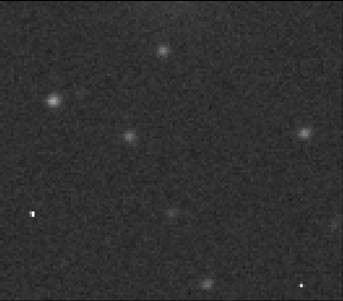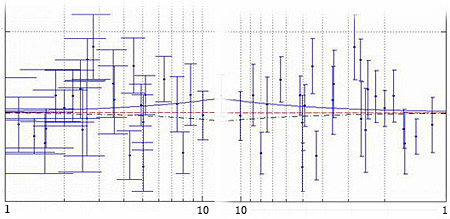# 标签存档： Matlab

## 误差棒函数1.1

% ERRORBARE Enhanced Errorbar Function.
% ERRORBARE(STY,X,Y,Xbar,Ybar,symbol)
% It can draw errorbar along X/Y/Dual axis
% in normal,semilog,loglog coordinate system,
% and adjust width of bar handles automatically,
% can also control dotstyle/color in the same way with errorbar.
%
% If the lower and upper error range of x/y is different,
% they should be input as [lower,upper] if x/y is a column vector;
% for a row vector, they should be [lower;uper].
%
% parameter STY include 12 types:
% v,h,d,vlogx,hlogx,dlogx,vlogy,hlogy,
% dlogy,vlogd,hlogd,dlogd
% where
% v stands for vertical errorbar，
% h draws horizontal errorbar，
% d means dual direction,
% logx corresponding to semilogx，can use preffix v/h/d
% logy corresponding to semilogy，can use preffix v/h/d
% logd corresponding to loglog，can use preffix v/h/d

## 用Matlab做星点识别## Matlab误差棒增强版

Matlab中自带的误差棒函数 errorbar 功能单一，只能在普通坐标下绘制纵向误差，而水平误差棒、对数坐标也经常会用到，就写了一个功能完整点的。帮助如下：

% 误差棒函数增强版
% ERRORBARE(SYM,X,Y,Xbar,Ybar) 能够按需要绘制误差棒，
% 可沿水平、垂直，或者两轴方向，能够根据所选坐标类型
% 调整端点线长。并可处理上下限不同的情况。
%
% SYM 参数包括 v,h,d,vlogx,hlogx,dlogx,vlogy,hlogy,
% dlogy,vlogd,hlogd,dlogd 共12种
% v 表示误差棒垂直，
% h 表示误差棒水平，
% d (dual) 显示双轴误差，
% logx 对应 semilogx，前缀 v,h,d 意义同上
% logy 对应 semilogy，前缀 v,h,d 意义同上
% logd 对应 loglog，前缀 v,h,d 意义同上
%
% 范例:
% x = 1:10;
% y = sin(x)+2;
% e = std(y)*ones(size(x));
% errorbare(x,y,e) % 直接调用errorbar函数
% errorbare(‘v’,x,y,e) % e为 y 误差限
% errorbare(‘v’,x,y,[e;2*e]) % 上下限不等
% errorbare(‘hlogx’,x,y,e) % e为 x 误差限，
% errorbare(‘d’,x,y,e,e) % 双轴误差棒

## Matlab之errorbar

tee = (max(x(:))-min(x(:)))/100;
% make tee .02 x-distance for error bars

xl = x – tee; 计算左端点
xr = x + tee; 右端点

xl = x/10^tee;
xr = x*10^tee;Update: